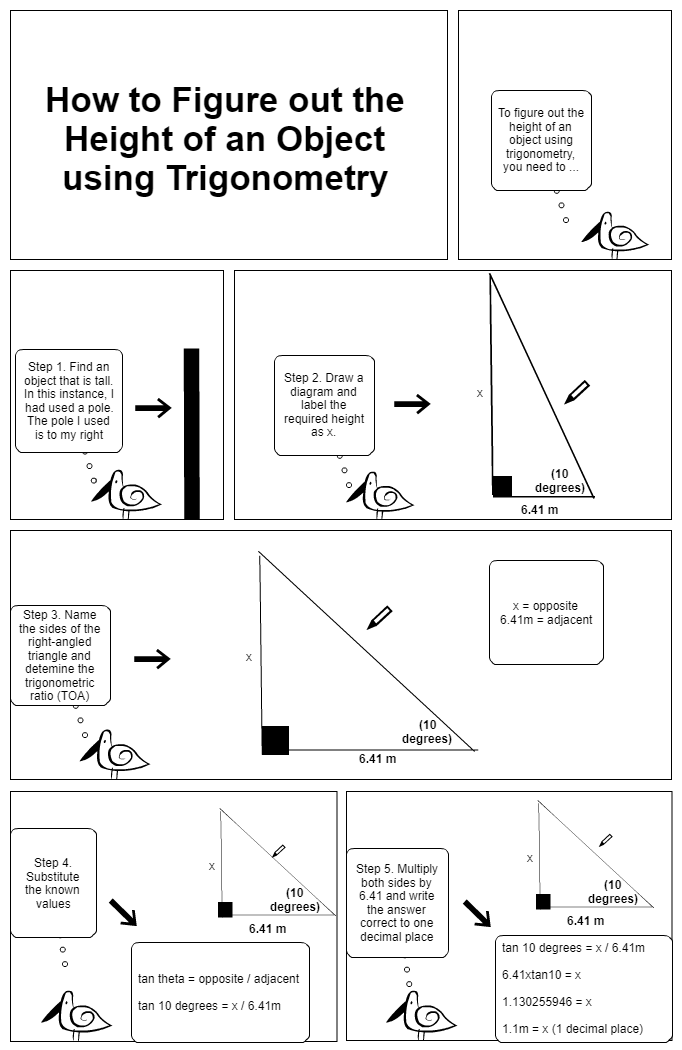Stripview

0 ThumbsUp

Height of an object using Trignonometry

anonymous on 12. Jun, 2019 — Lang: English• Transcript

Expand

How to Figure out the Height of an Object using Trigonometry

To figure out the height of an object using trigonometry, you need to ...

Step 1. Find an object that is tall.
In this instance, I had used a pole.
The pole I used is to my right

(10 degrees)

6.41 m

x

Step 3. Name the sides of the right-angled triangle and detemine the trigonometric ratio (TOA)

(10 degrees)

6.41 m

x

x = opposite

Step 4. Substitute the known values

(10 degrees)

6.41 m

x

Step 5. Multiply both sides by 6.41 and write the answer correct to one decimal place

(10 degrees)

6.41 m

x

Step 2. Draw a diagram and label the required height as x.

tan theta = opposite / adjacent

tan 10 degrees = x / 6.41m

tan 10 degrees = x / 6.41m

6.41xtan10 = x

1.130255946 = x

1.1m = x (1 decimal place)

Tags:
#maths